# Solutions

As mentioned within the session setup, load the following packages using the `library()` function. Additionally, as we will be using a data set with large numbers, set scipen to 999 using the option function.

``````  library(tidyverse)
library(RColorBrewer)

options(scipen = 999)``````

As mentioned previously, for the purpose of this session, we will be using a sample of the Online Gaming Anxiety Data, this subset is avaliable to download as part of the .zip file downloadable from the Github Repo. It can then be loaded using the following script:

``gamingdata_samp <- read_csv("data/gamingdata_samp.csv")``

Section 1: ggplot2 vs plot

Exercise 1: Plotting SPIN_T against Age using both the `plot()` and `ggplot()` function, discuss which has more potential in displaying data clearly.

``````## Plotting with plot()
plot(data = gamingdata_samp,
SPIN_T ~ Age)````````````## Plotting with ggplot()

ggplot(data = gamingdata_samp,
mapping = aes(x = Age,
y = SPIN_T)) +
geom_point()``````
``## Warning: Removed 250 rows containing missing values (geom_point).``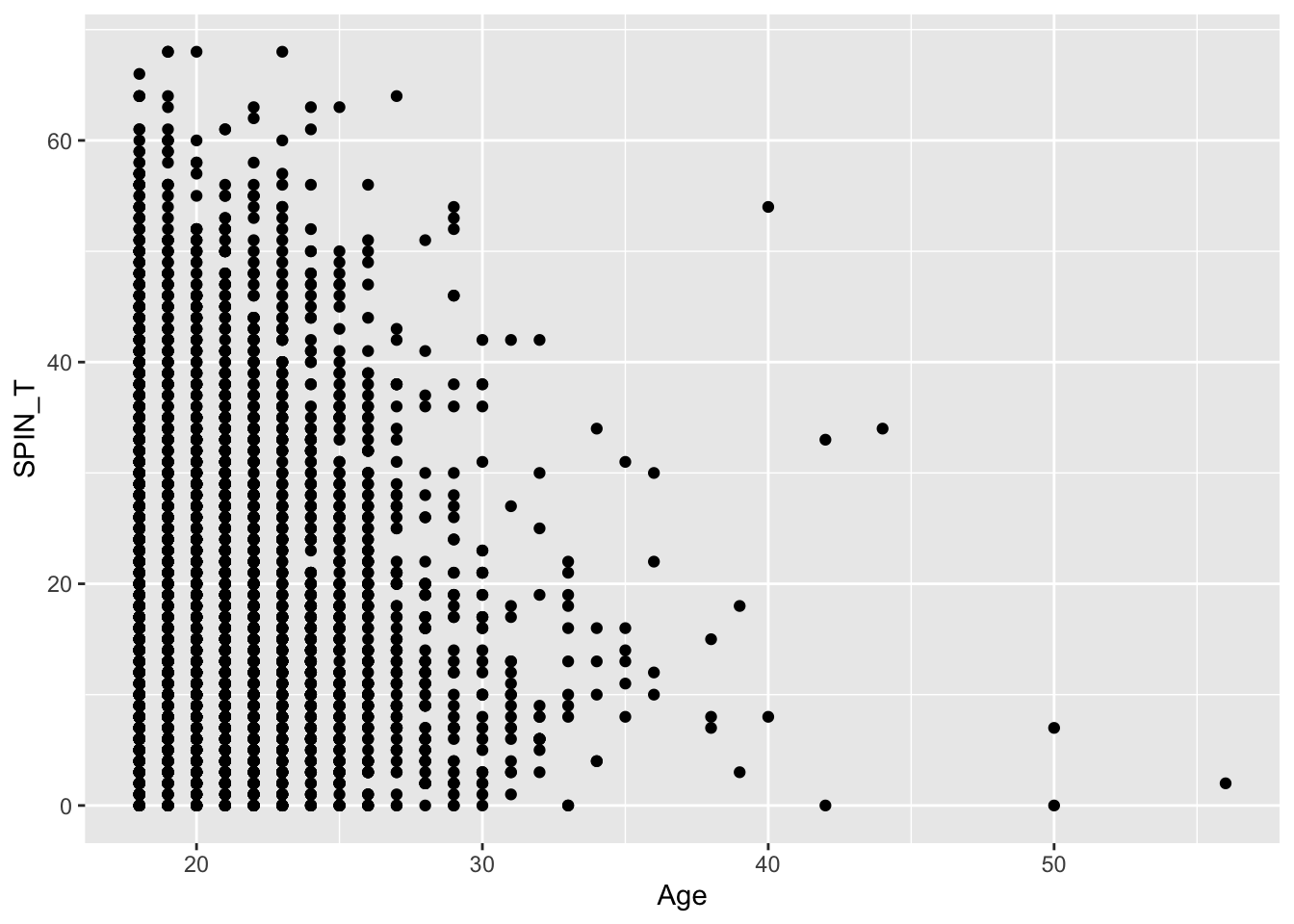Exercise 2: Expand the plot to group these points by Gender, which provides us with more information and is easier to achieve? Remember, you’ll need to recode gamingdata_samp\$Gender as a factor using the function:

``````  gamingdata_samp\$Gender <- as.factor(gamingdata_samp\$Gender)

plot(data = gamingdata_samp,
SPIN_T ~ Age, col = c("blue", "red")[Gender])``````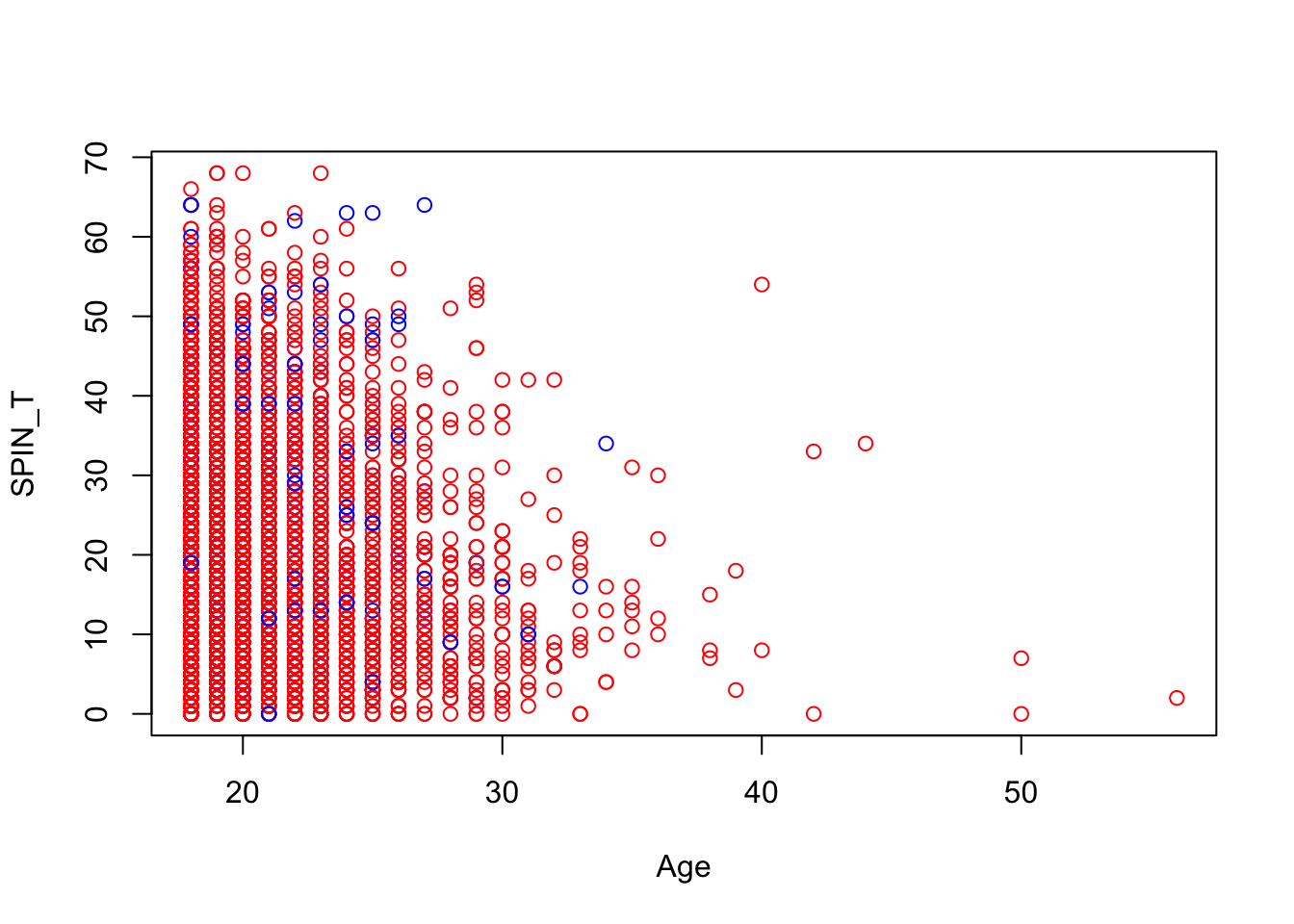``````  ggplot(data = gamingdata_samp,
mapping = aes(x = Age,
y = SPIN_T,
colour = Gender)) +
geom_point()``````
``## Warning: Removed 250 rows containing missing values (geom_point).``Section 2: Basic Plotting Using ggplot

Exercise 3: Change the size parameter to Hours to see if there is a trend between the amount of hours, age and total SPIN score.

``````  ggplot(data = gamingdata_samp,
mapping = aes(x = Age,
y = SPIN_T,
colour = Gender,
size = Hours)) +
geom_point()``````
``## Warning: Removed 261 rows containing missing values (geom_point).``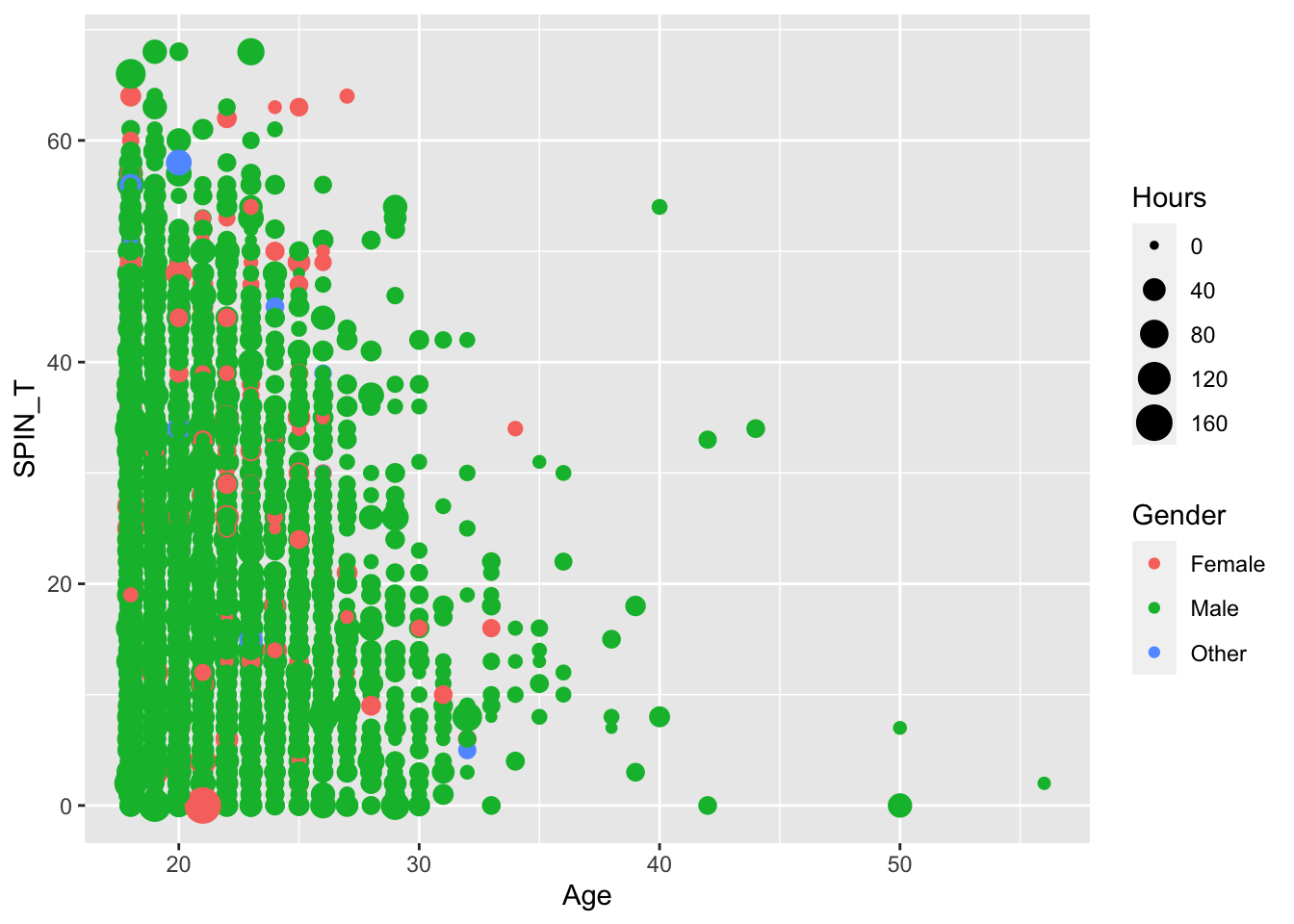Exercise 4: Change the geom, from geom_point() to geom_jitter() and change the alpha parameter to 0.5 to more clearly see the relationship

``````  ggplot(data = gamingdata_samp,
mapping = aes(x = Age,
y = SPIN_T,
colour = Gender,
size = Hours)) +
geom_jitter(alpha = 0.5)``````
``## Warning: Removed 261 rows containing missing values (geom_point).``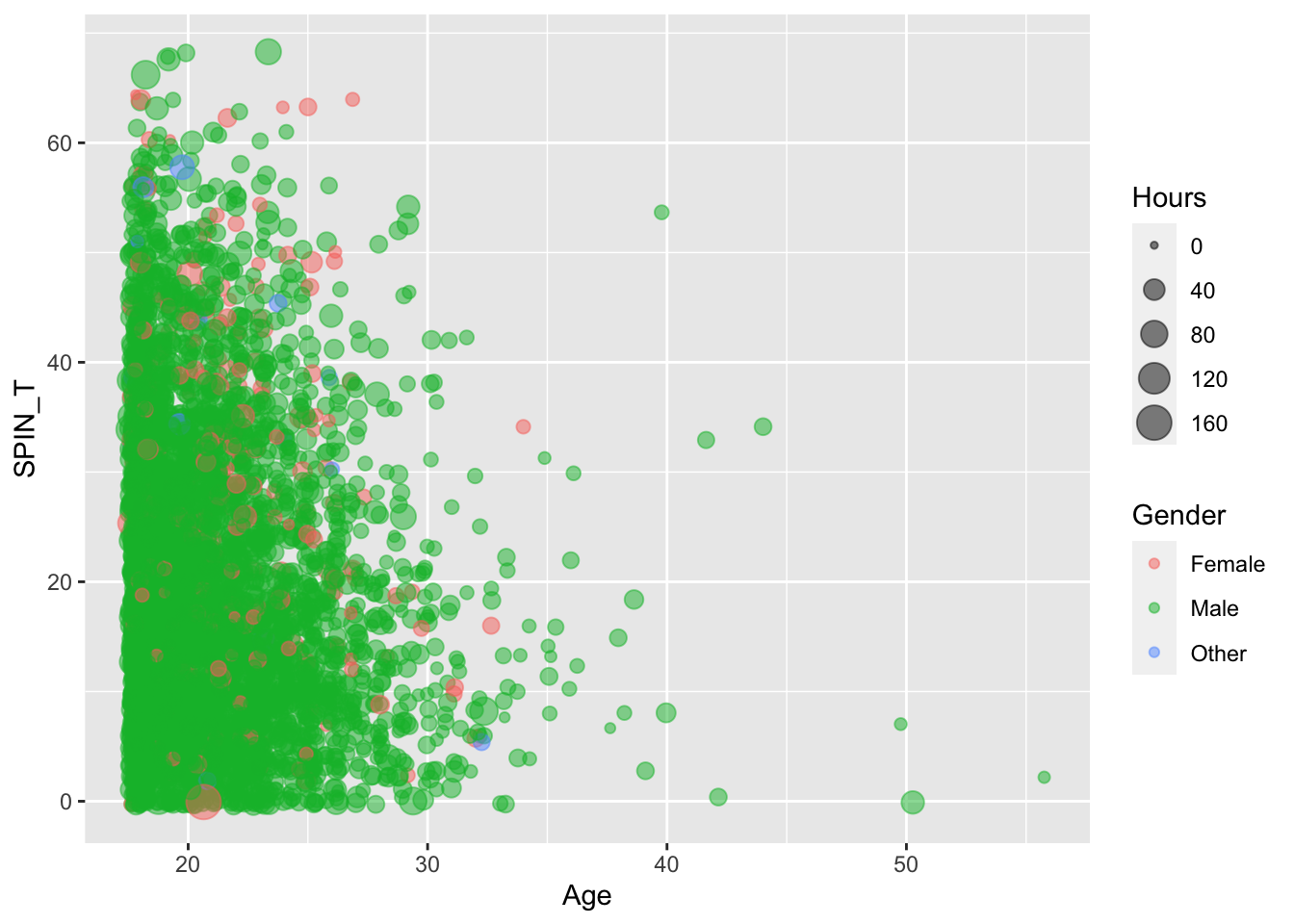Exercise 5: Change the X and Y axis labels, and title to something suitable for the plot, using the the labs() addition

``````  ggplot(data = gamingdata_samp,
mapping = aes(x = Age,
y = SPIN_T,
colour = Gender,
size = Hours)) +
geom_jitter(alpha = 0.5) +
labs(x = "Age", y = "SPIN Total Score",
title = "Age in Relation to SPIN Total Score")``````
``## Warning: Removed 261 rows containing missing values (geom_point).``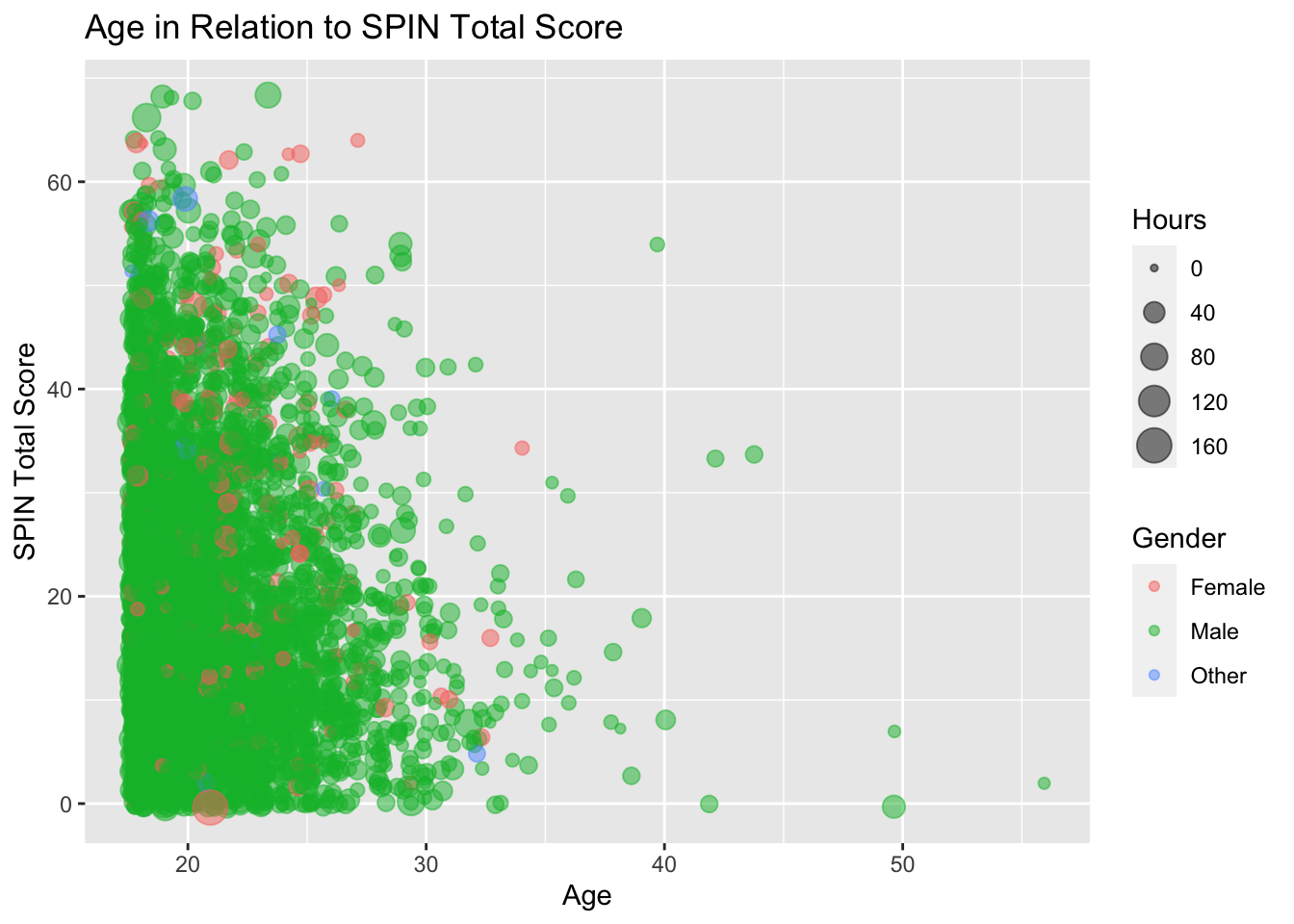Section 3: Different geom’s different plots

Exercise 6: Using geom_bar() plot the number of different cases in each gender

``````  ggplot(data = gamingdata_samp,
mapping = aes(x = Gender)) +
geom_bar()``````Exercise 7: Expand upon the geom_bar() plot, to determine the average (mean) number of Hours played by each gender.

``````  ggplot(data = gamingdata_samp,
mapping = aes(x = Gender, y = Hours)) +
geom_bar(stat = "summary")``````
``## Warning: Removed 11 rows containing non-finite values (stat_summary).``
``## No summary function supplied, defaulting to `mean_se()```Exercise 8: Expanding again on the plot created in Exercise 6, after using the following code to convert the Platform variable into a factor, specify the fill value to Platform.

``````  gamingdata_samp\$Platform <- as.factor(gamingdata_samp\$Platform)

ggplot(data = gamingdata_samp,
mapping = aes(x = Gender, fill = Platform)) +
geom_bar()``````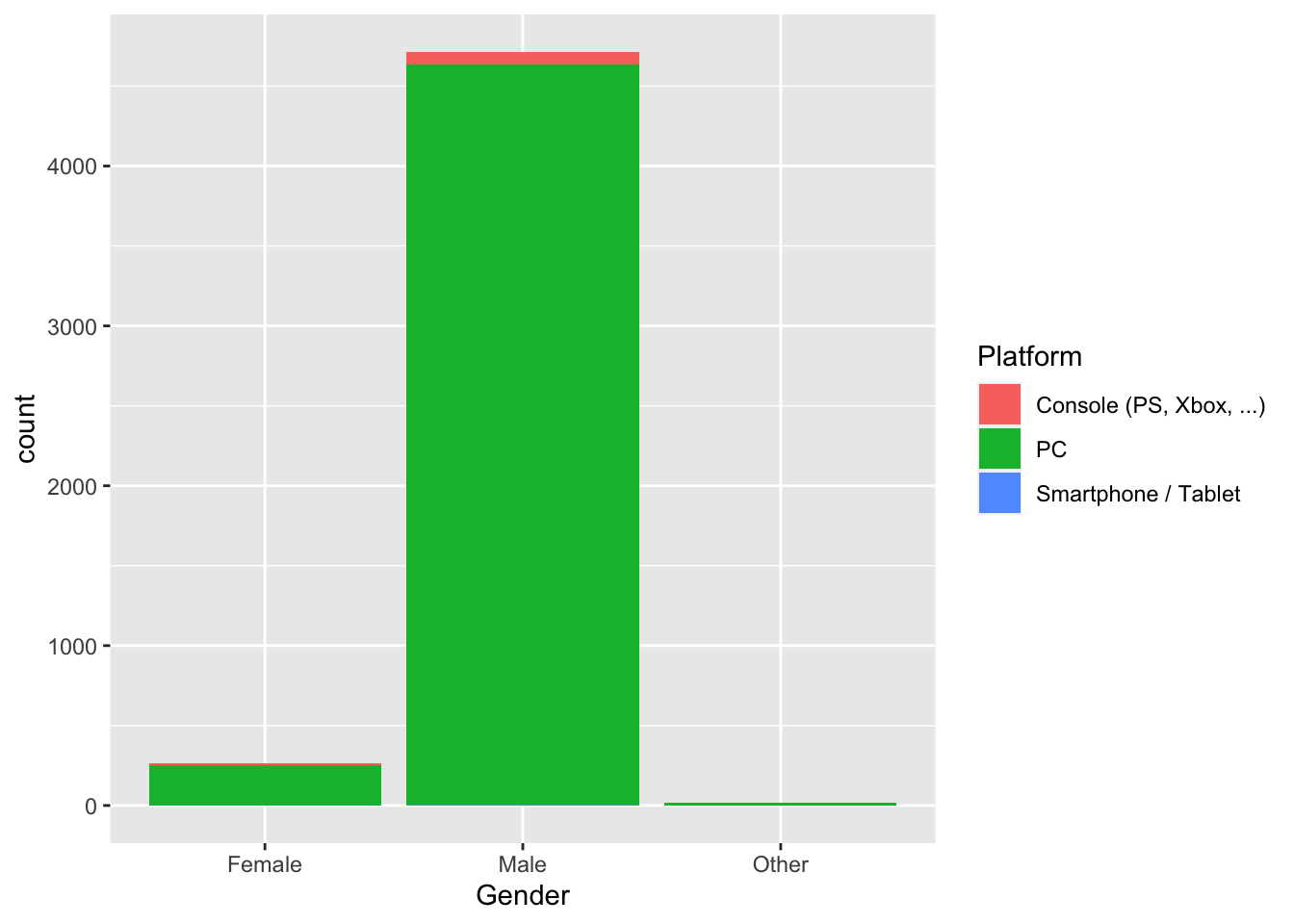Exercise 9: Through adding the appropriate limits function (xlim(), ylim(), lims()) in ggplot() set the upper threshold of your plot to 5,000

``````  gamingdata_samp\$Platform <- as.factor(gamingdata_samp\$Platform)

ggplot(data = gamingdata_samp,
mapping = aes(x = Gender, fill = Platform)) +
geom_bar() +
ylim(0, 5000)``````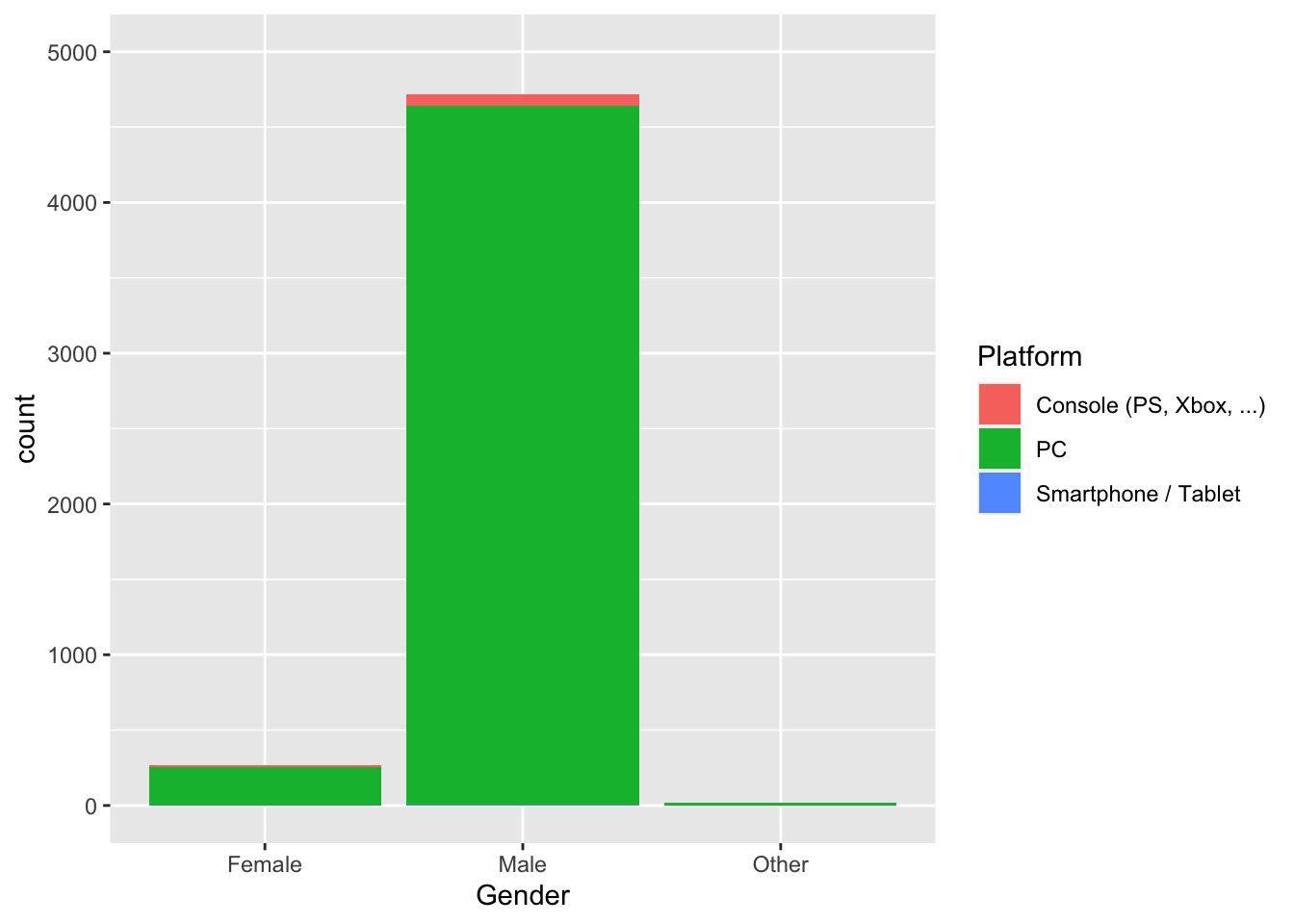Section 4: Customization Part 1

Exercise 10: Using all the customization skills we have already discussed (labs(), lims() and geom’s), examine the relationship between an individuals Age, Number of Hours playing, their employment and what platform they use. Once completed, add one of the following themes to the end of your code, which looks best (to you)?

• theme_bw()
• theme_minimal()
• theme_void()
• theme_dark()
• theme_classic

Hint: Consider using the mapping aesthetics such as colour, size, shape, alpha etc, to map the categorical variables.

``````ggplot(data = gamingdata_samp,
mapping = aes(x = Age, y = Hours,
colour = Platform, shape = Work)) +
geom_point(alpha = 0.5) +
labs(x = "Age", y = "Hours Played") +
lims(x = c(18, 60), y = c(0, 200)) +
theme_minimal()``````
``## Warning: Removed 24 rows containing missing values (geom_point).``Section 5: Layering different geom’s

Exercise 11: Using the code developed in Section 2 (Age, Hours and Total Spin Score) add an additional geom_jitter() layer which plots the GAD score.

``````  ggplot(data = gamingdata_samp) +
geom_jitter(alpha = 0.5,
mapping = aes(x = Age,
y = SPIN_T,
colour = Gender,
size = Hours)) +
geom_jitter(alpha = 0.5,
mapping = aes(x = Age,
colour = Gender,
size = Hours)) +
labs(x = "Age", y = "SPIN Total Score",
title = "Age in Relation to SPIN Total Score")``````
``## Warning: Removed 261 rows containing missing values (geom_point).``
``## Warning: Removed 11 rows containing missing values (geom_point).``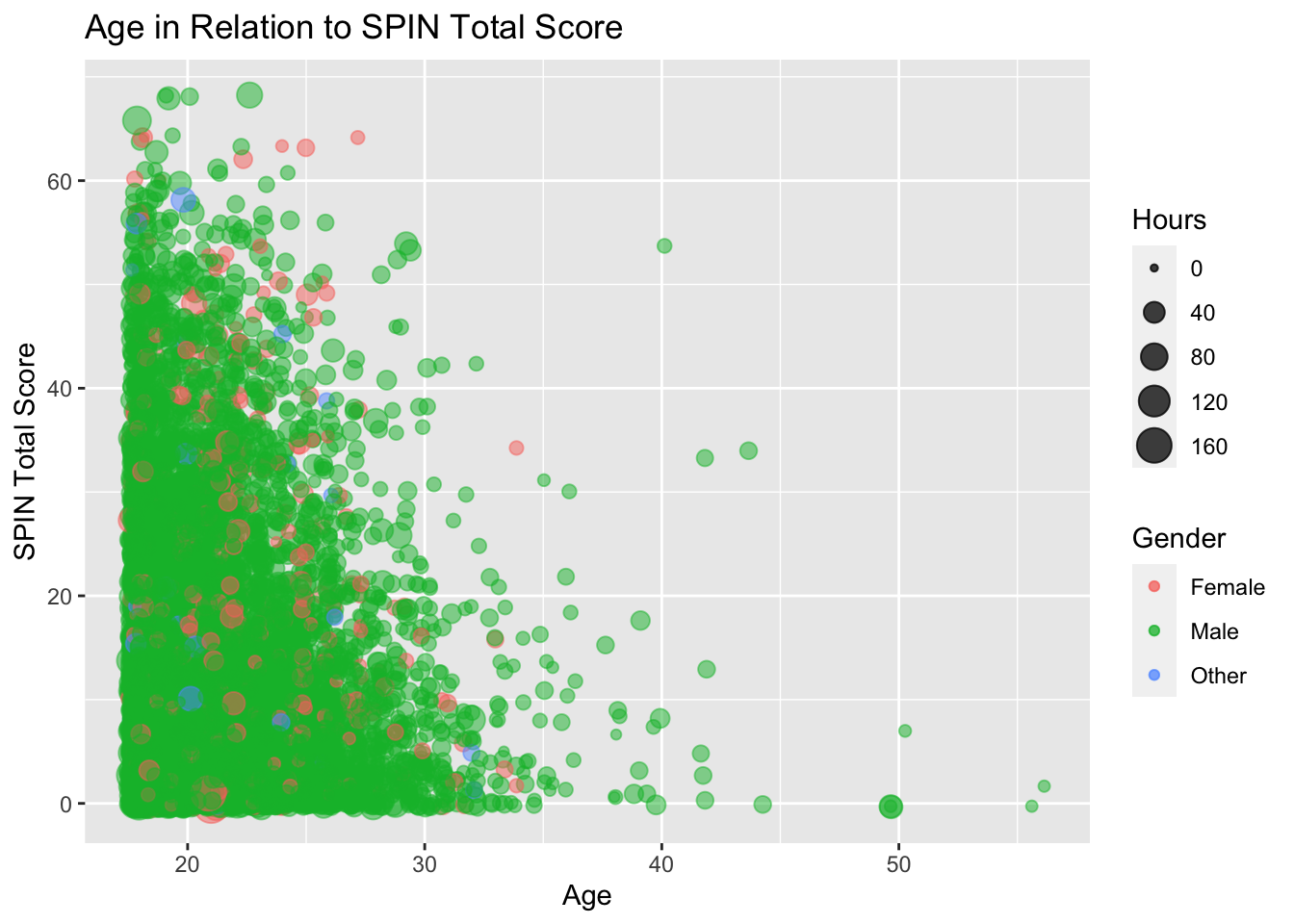Exercise 12: Expanding on the code from Exercise 11, replace the colour variable (currently Gender) with a colour to differentiate between SPIN and GAD. You can add back in Gender as a shape variable (see here for options).

``````  ggplot(data = gamingdata_samp) +
geom_jitter(alpha = 0.5,
mapping = aes(x = Age,
y = SPIN_T,
colour = "red",
size = Hours,
shape = Gender)) +
geom_jitter(alpha = 0.5,
mapping = aes(x = Age,
colour = "blue",
size = Hours,
shape = Gender)) +
labs(x = "Age", y = "SPIN Total Score",
title = "Age in Relation to SPIN Total Score")``````
``## Warning: Removed 261 rows containing missing values (geom_point).``
``## Warning: Removed 11 rows containing missing values (geom_point).``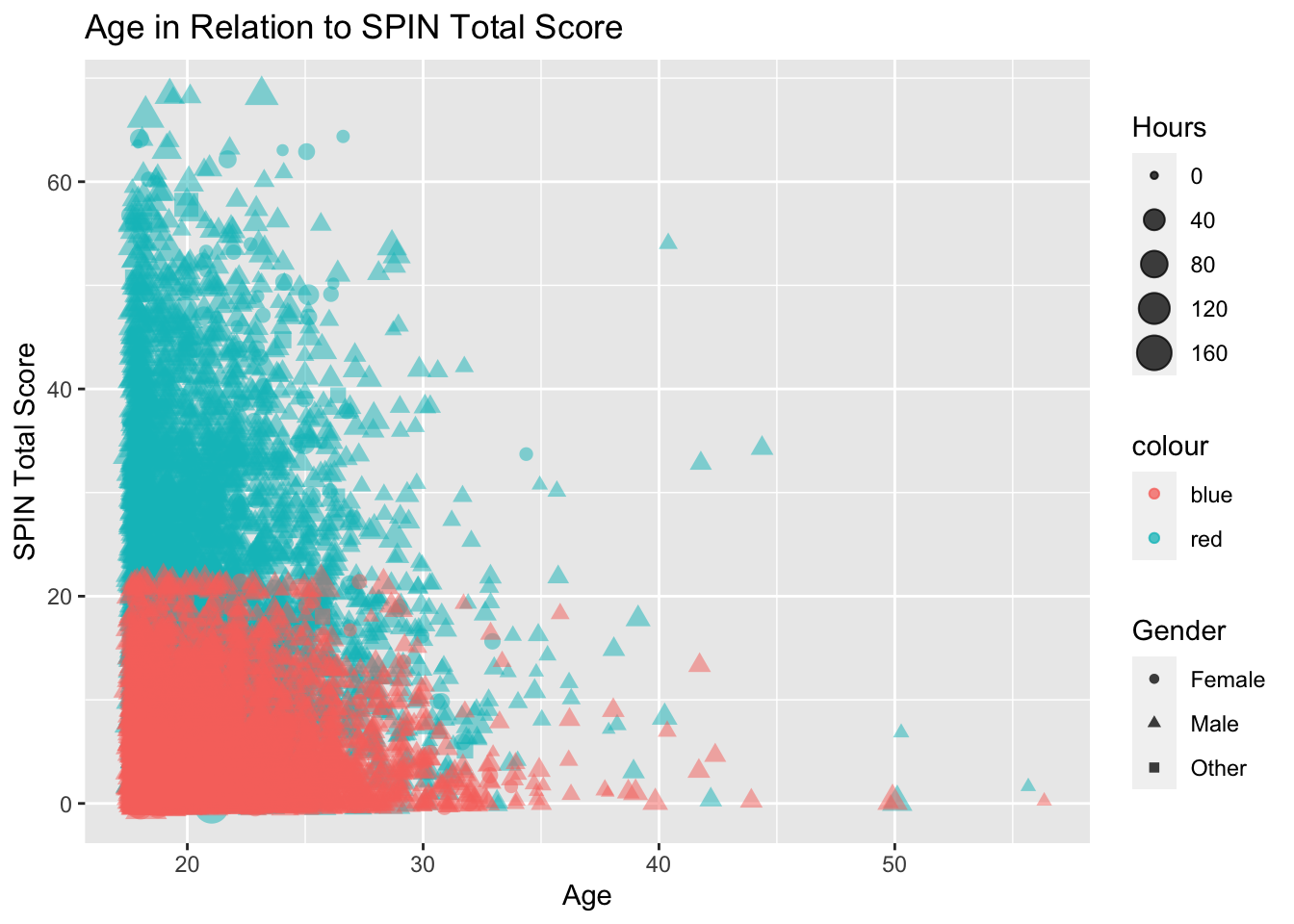Exercise 13: Conducting a direct swap between a colour (“blue” or “red”) and the variable Gender, does not produce a usable legend. As such, remove the colour parameter from the mapping = aes() component.

``````  ggplot(data = gamingdata_samp) +
geom_jitter(alpha = 0.5,
mapping = aes(x = Age,
y = SPIN_T,
size = Hours,
shape = Gender),
colour = "red") +
geom_jitter(alpha = 0.5,
mapping = aes(x = Age,
size = Hours,
shape = Gender),
colour = "blue") +
labs(x = "Age", y = "SPIN Total Score",
title = "Age in Relation to SPIN Total Score")``````
``## Warning: Removed 261 rows containing missing values (geom_point).``
``## Warning: Removed 11 rows containing missing values (geom_point).``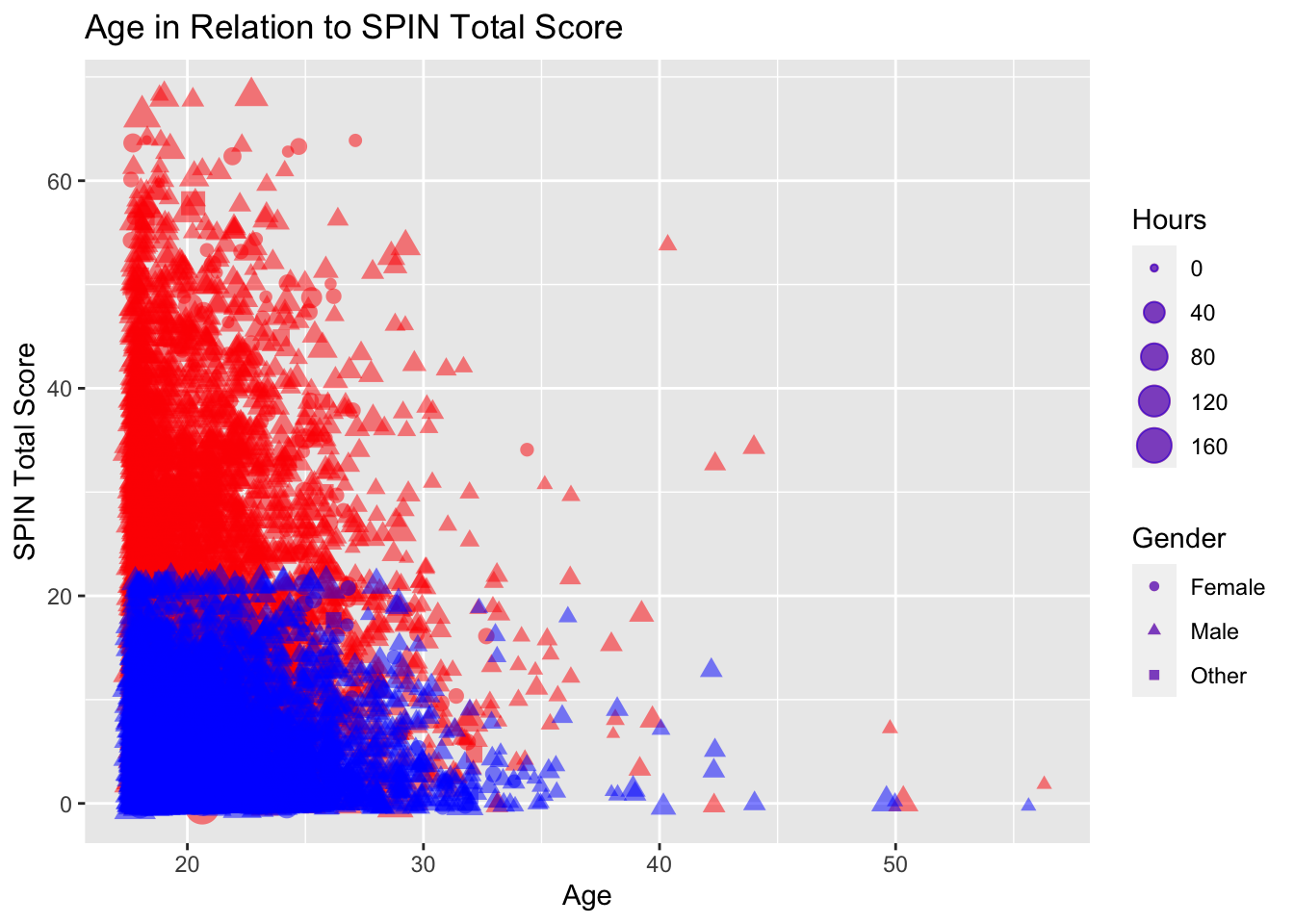Exercise 13: Although this corrects the colours, there is no usable legend. To add a colour specific legend, you must return the colour = parameter to inside mapping=aes(), alongside adding scale_colour_identity(). To apply this, use the given template to specify what the colours indicates. Additionally correct the axis names so they represent the contents of the plot.

`````` ggplot(data = gamingdata_samp) +
geom_jitter(alpha = 0.5,
mapping = aes(x = Age,
y = SPIN_T,
size = Hours,
shape = Gender,
colour = "red")) +
geom_jitter(alpha = 0.5,
mapping = aes(x = Age,
size = Hours,
shape = Gender,
colour = "blue")) +
labs(x = "Age", y = "Total Anxiety Measure Score",
title = "Age in Relation to SPIN Total Score") +
scale_colour_identity(name = "Anxiety Measure",
breaks = c("red", "blue"),
guide = "legend")``````
``## Warning: Removed 261 rows containing missing values (geom_point).``
``## Warning: Removed 11 rows containing missing values (geom_point).``Exercise 14: Colours and legends can also be used for continuous variables. Return to just a single variable (SPIN_T), and specify the colour parameter as the hours variable (Hours). Is this clearer than the when Hours was specified as the size variable?

`````` ggplot(data = gamingdata_samp) +
geom_jitter(alpha = 0.5,
mapping = aes(x = Age,
y = SPIN_T,
shape = Gender,
colour = Hours)) +
labs(x = "Age", y = "Total Anxiety Measure Score",
title = "Age in Relation to SPIN Total Score")``````
``## Warning: Removed 250 rows containing missing values (geom_point).``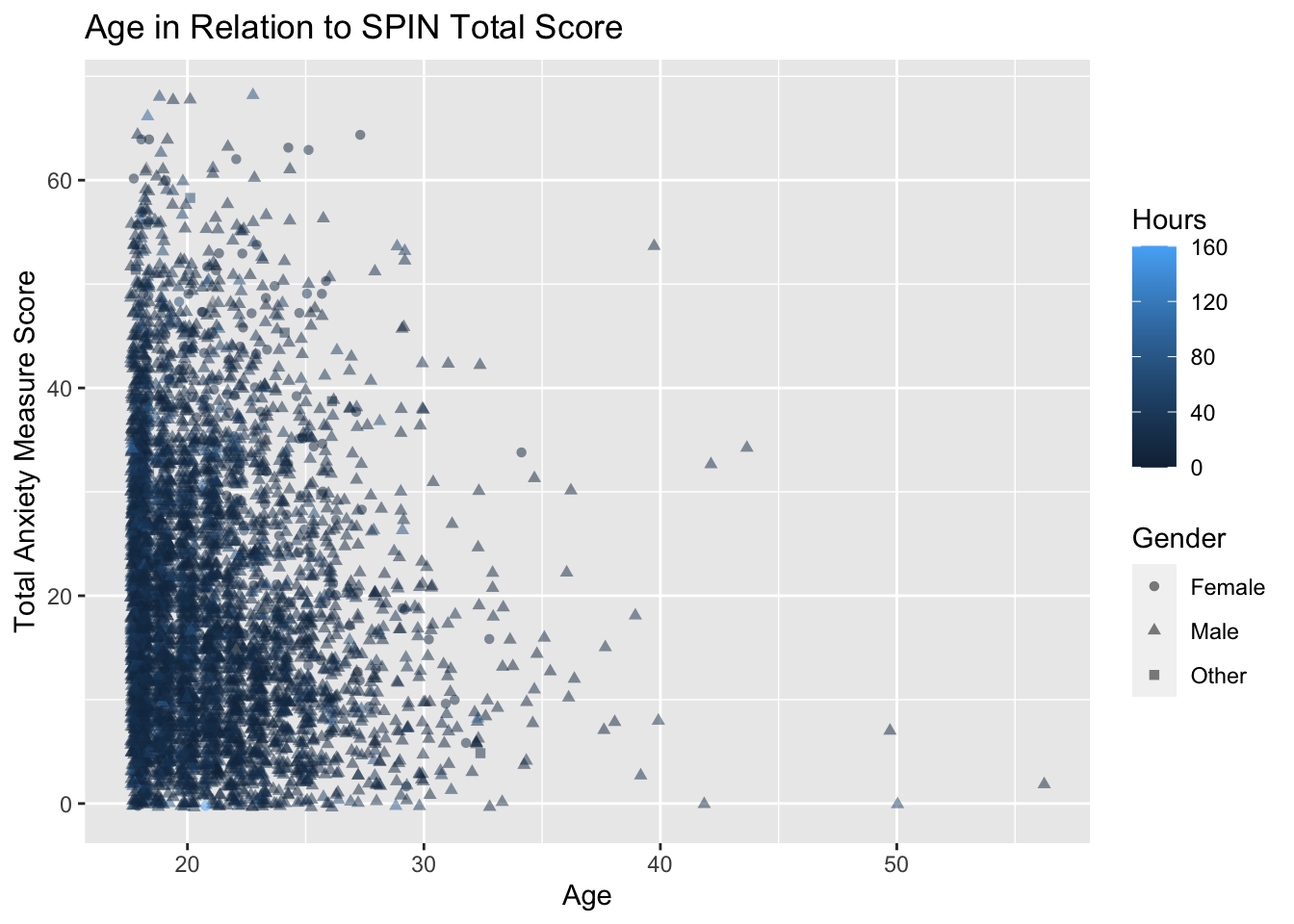Exercise 15: To improve the clarity of the scale, you can use the function scale_colour_gradient() to specify the colours of the low and high points as well as any specific breaks. Add this function to the previous plot (Ex.14), using the template provided. Think about what are good breaks to use within this function.

`````` ggplot(data = gamingdata_samp) +
geom_jitter(alpha = 0.5,
mapping = aes(x = Age,
y = SPIN_T,
shape = Gender,
colour = Hours)) +
labs(x = "Age", y = "Total Anxiety Measure Score",
title = "Age in Relation to SPIN Total Score") +
scale_colour_gradient(low = "Yellow", high = "Red",
breaks = c(0, 50, 100, 150))``````
``## Warning: Removed 250 rows containing missing values (geom_point).``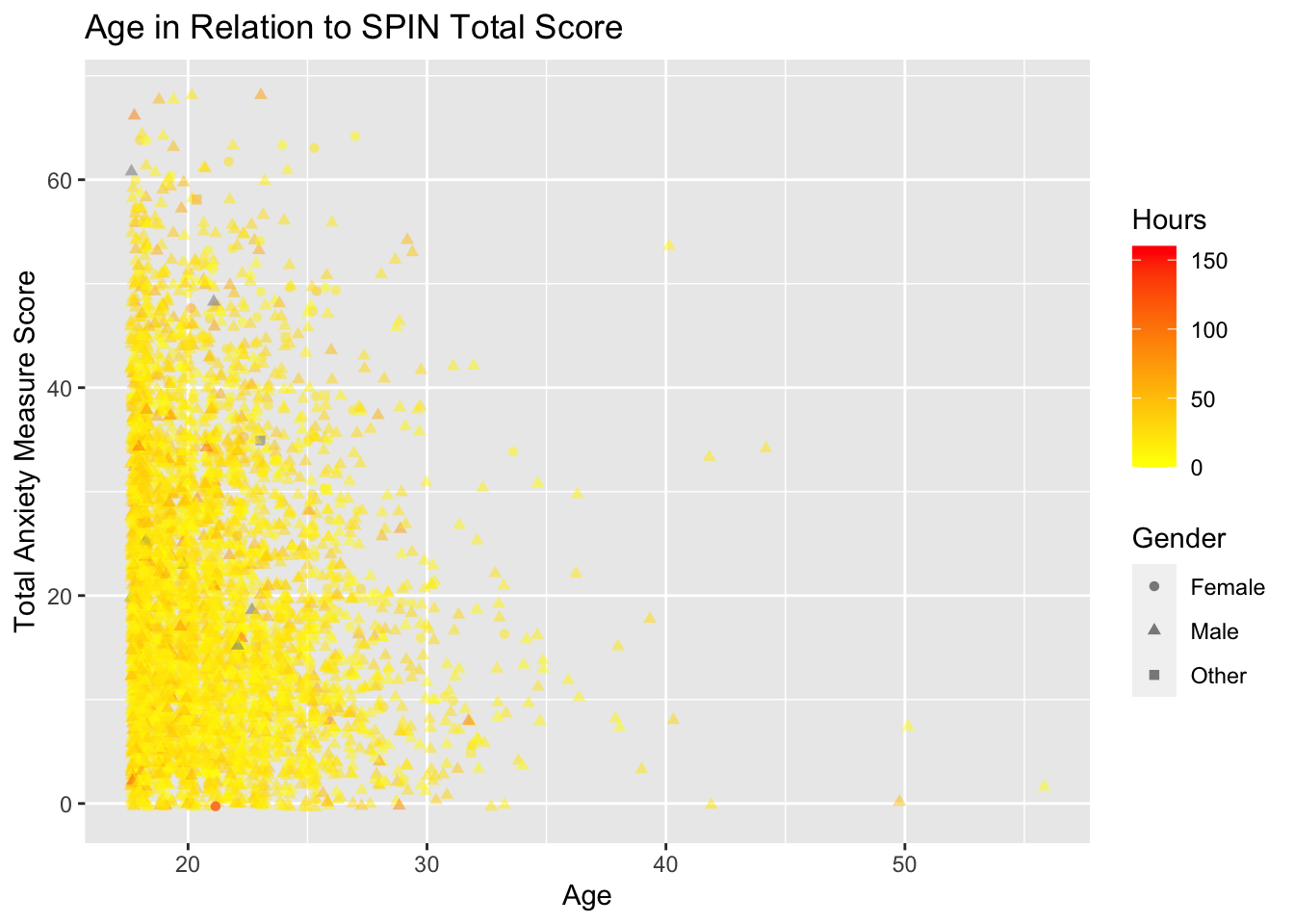Exercise 16: An alternative to layering multiple plots on on top of each other, is to use facet_wrap() or facet_grid() to produce graphs side by side. Using this a faceting function you can split the graphs up by a specific variable. Try using facet_wrap() to split the graph produced in Ex.13, by Gender.

`````` ggplot(data = gamingdata_samp) +
geom_jitter(alpha = 0.5,
mapping = aes(x = Age,
y = SPIN_T,
size = Hours,
shape = Gender,
colour = "red")) +
geom_jitter(alpha = 0.5,
mapping = aes(x = Age,
size = Hours,
shape = Gender,
colour = "blue")) +
labs(x = "Age", y = "Total Anxiety Measure Score",
title = "Age in Relation to SPIN Total Score") +
scale_colour_identity(name = "Anxiety Measure",
breaks = c("red", "blue"),
guide = "legend") +
facet_grid(.~ Gender)``````
``## Warning: Removed 261 rows containing missing values (geom_point).``
``## Warning: Removed 11 rows containing missing values (geom_point).``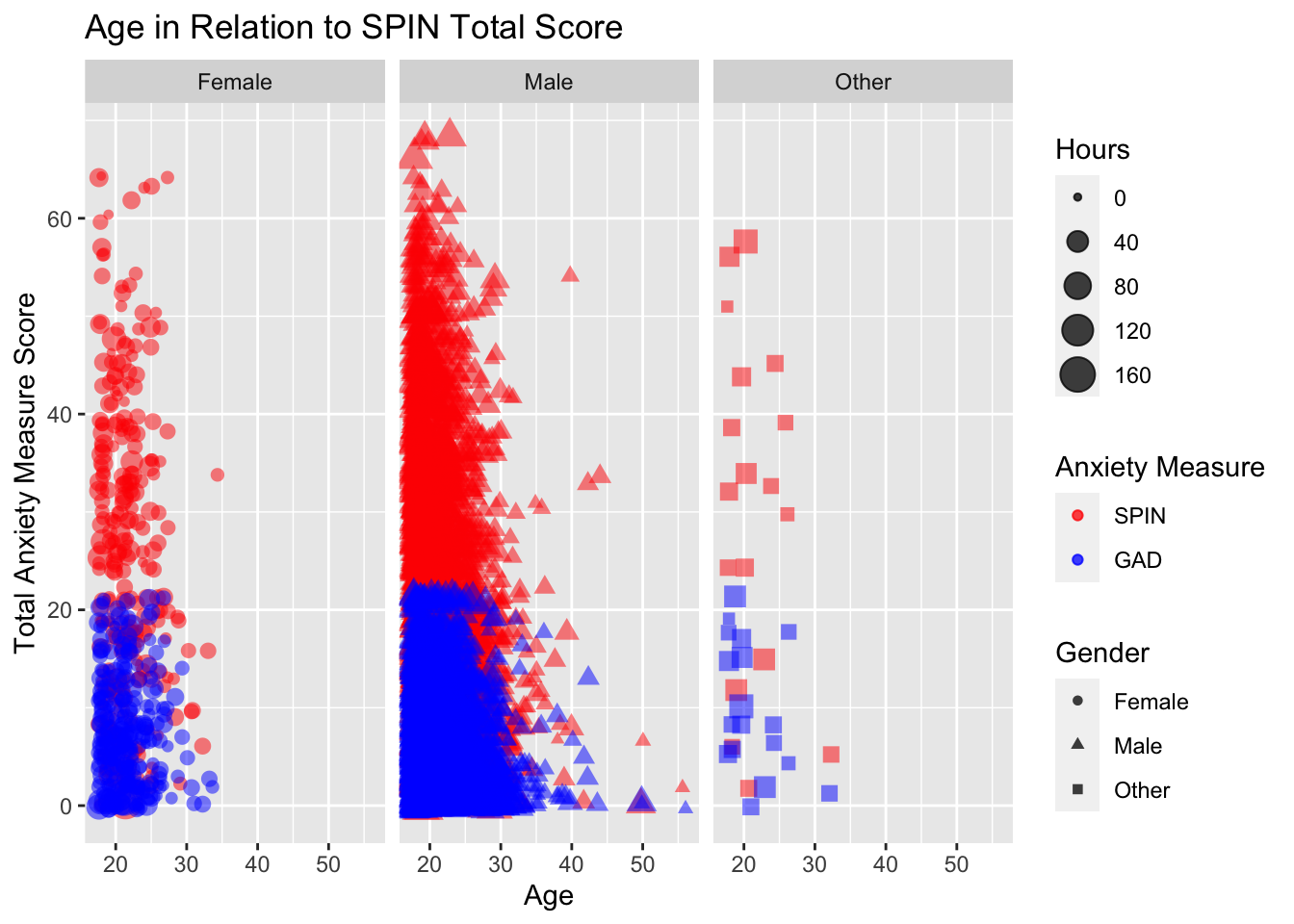Exercise 17. Alternatively you can split by a factor not included in the graph at the point of creation. Try using facet_grid() again to this time split the same graph by “Platform”.

`````` ggplot(data = gamingdata_samp) +
geom_jitter(alpha = 0.5,
mapping = aes(x = Age,
y = SPIN_T,
size = Hours,
shape = Gender,
colour = "red")) +
geom_jitter(alpha = 0.5,
mapping = aes(x = Age,
size = Hours,
shape = Gender,
colour = "blue")) +
labs(x = "Age", y = "Total Anxiety Measure Score",
title = "Age in Relation to SPIN Total Score") +
scale_colour_identity(name = "Anxiety Measure",
breaks = c("red", "blue"),
guide = "legend") +
facet_grid(.~ Platform)``````
``## Warning: Removed 261 rows containing missing values (geom_point).``
``## Warning: Removed 11 rows containing missing values (geom_point).``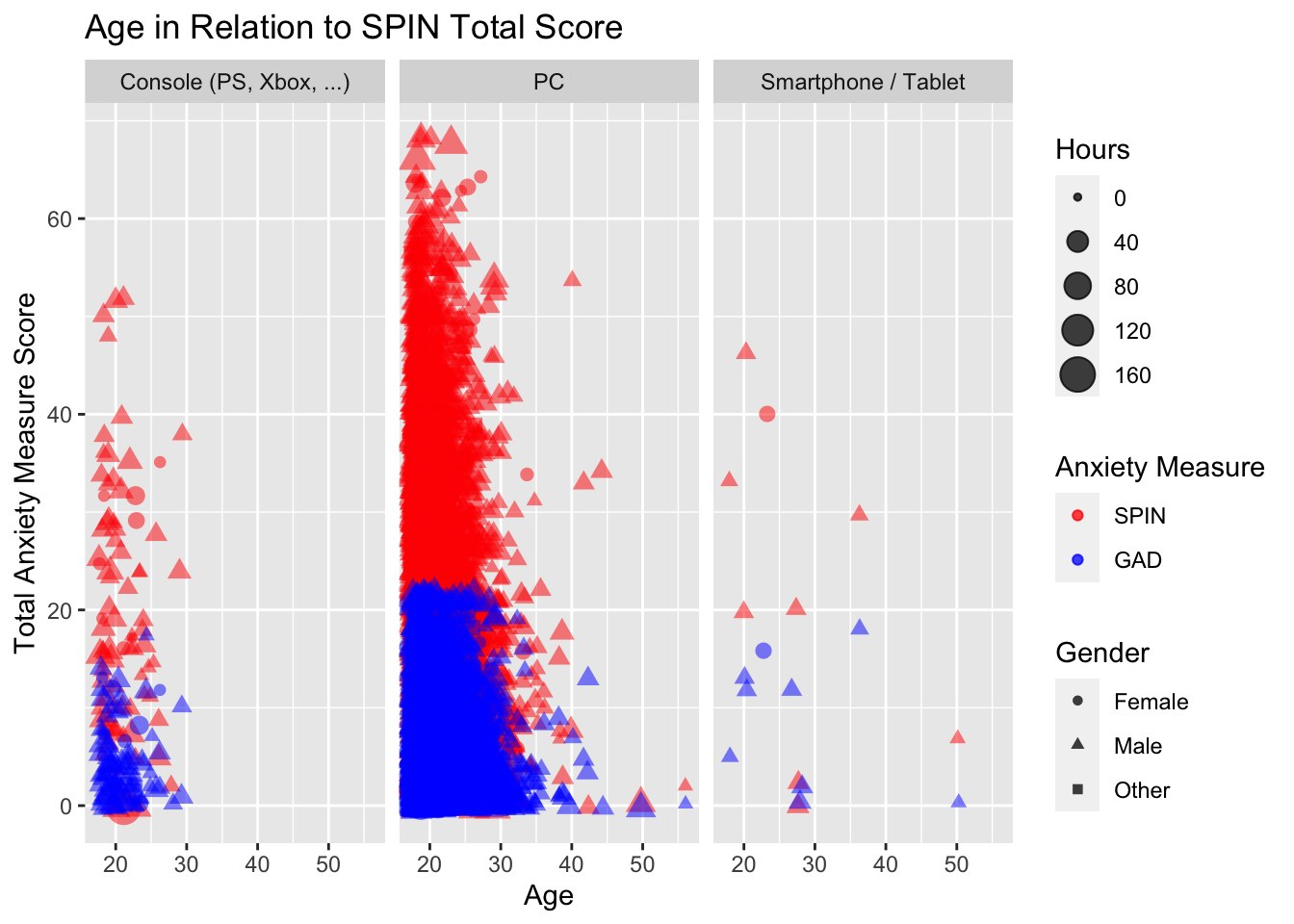Section 6: Publishing Plots from ggplot2

Exercise 18: Using the function ggsave(), export your last plot (using the parameter last_plot()). Use the template provided to structure your export.

``````ggsave(filename = "test_plot.png",
plot = last_plot())``````

Exercise 19: Alternatively, you can set a specific graph to a variable (for example: “plot_to_print”) and past it in place of last_plot(). Additionally, you can change parameters such as the height and width, dpi, scale and type of export accordingly. Try to export the plot you made for Ex.15, as a JPEG (.jpeg), with a dpi of 500 and a size of 5cmx10cm.

``````ggsave(filename = "test_plot2.jpeg",
plot = plot_to_print,
height = 5,
width = 10,
dpi = 500)``````

Exercise 20: Alternatively, you can also use the export function in the Rstudio UI to export your plots. To do this, click export in your plots tab (if plots are produced there) and export accordingly. If you are using the accompanying worksheet (which is a .R script), try saving any of your previous plots as .pdf files following the instructions in RStudio.